Courses
Courses for Kids
Free study material
Free LIVE classes
More

# Divide Multi-Digit Numbers Worksheet## A Brief Explanation of the Divide Multi-Digit Numbers Worksheet

Do you ever realise the importance of dividing a number in everyday life? If so, then you might be wondering about what the division is. Division is the basic arithmetic operation used in maths to divide a group of things into equal parts. There are 4 parts to know about when using the concept of division, i.e. dividend, divisor, quotient, and remainder. Let’s see more about the division and 2-digit division worksheets, along with 4-digit by 2-digit division worksheets, in the article given below.

## Explain the Division of Two Numbers

Division is the tool used to distribute objects equally among different groups of people. The dividend is the number being divided; the divisor is the number by which the dividend is being divided; the quotient is the result of division, and the remainder is the amount left after the division.

## Steps of Division

The following steps discuss the process of division:

• Step 1: Take the first digit of the dividend from the left. Check if this digit is greater than or equal to the divisor.

• Step 2: Divide it by the divisor and write the answer on top as the quotient.

• Step 3: Subtract the result from the digit and write the difference below.

• Step 4: Bring down the next digit of the dividend (if present).

• Step 5: Repeat the same process.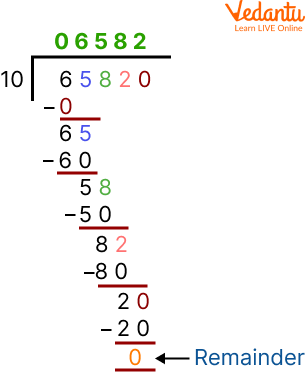Division

## Solved Examples of Dividing by 2-Digit Numbers Worksheet

In the following module, we will solve some examples based on the dividing by 2-digit number worksheet:

Q 1. Divide 94 by 12.

Ans: Given the dividend is 94, and the divisor is 12

We need to calculate the quotient and remainder. For that, one should divide the number 94 by 12.

Select the least number in the multiple of 12, which is near 94 or nearly equal to it.

Here, the number 12 is multiplied by the number 7 to equal to 84, and note the difference between 94 and 84, which is equal to 10 with a quotient of 7.

Thus, the quotient and remainder of a given division problem are 7 and 10, respectively.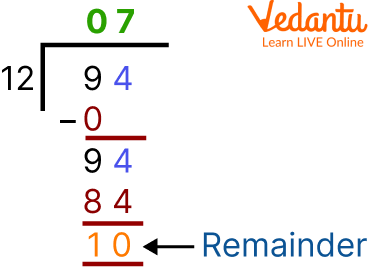Division of 94 by 12

Q 2. Divide 514 by 32.

Ans: Given the dividend is 514, and the divisor is 32

The number 32 is first multiplied by 1 so that it equals 32. Then, subtract 32 from the dividend; 51 - 32 = 19.

Now, the new dividend is 194. The number 32 is then multiplied by 6 to obtain 192. Take the difference between 194 and 192, i.e. 194 - 192 = 2.

The difference, 2, is less than the divisor, hence called the remainder.

Thus, the quotient and remainder of a given division problem are 16 and 2, respectively.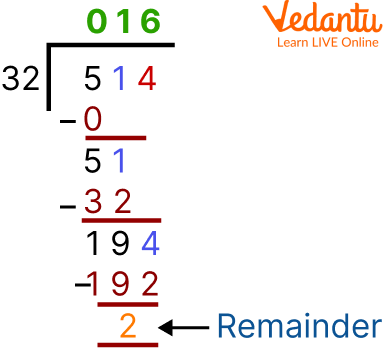Division of 514 by 32

Q 3. Divide 4963 by 14.

Ans: Given the dividend is 4963, and the divisor is 14

The number 14 is first multiplied by 3 so that it equals 42. Now, take the difference between 49 and 42, i.e. 49 - 42 = 7.

Consider the use of 76. Again, 14 is multiplied by 5 so that it equals 70. Then take the difference between 76 and 70, which equals 6.

63 becomes the dividend, so we multiply 14 by 4 to equal 56. Taking the difference between 63 and 56, we get 7, which is less than the divisor, hence called the remainder.

Thus, the quotient and remainder of the given division problem are 354 and 7, respectively.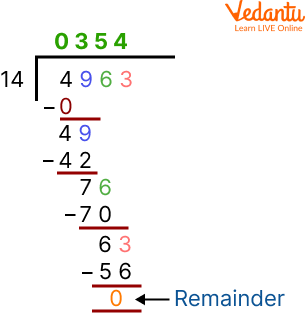Division of 4963 by 14

##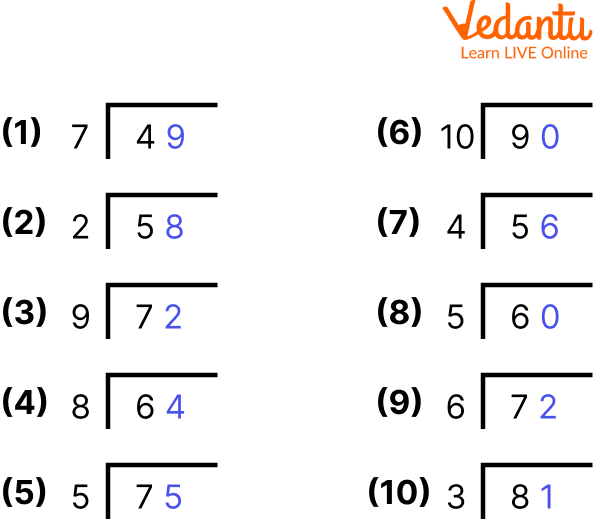2-Digit Division Worksheets

##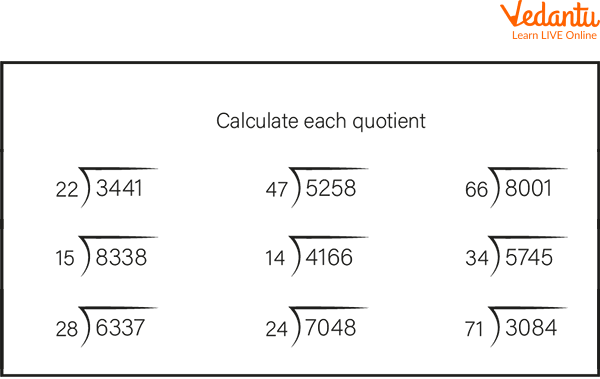4-Digit By 2-Digit Division Worksheets

## Long Division Problems for Practice

Q 1. Divide 200 by 50.

Ans: 4

Q 2. Find the quotient and remainder when 1550 is divided by 25.

Ans: Quotient = 62, Remainder = 0

Q 3. Compute the remainder when 257 is divided by 15.

Ans: Remainder = 2

## Summary

To sum up here with the topic of dividing multi-digit numbers worksheets. Division is an important tool for performing calculations in mathematics. The four parts of a division expression are divisor, dividend, remainder, and quotient. This module covers the division of two numbers and the steps for dividing, along with some solved examples of dividing by 2-digit numbers worksheets and long division problems for practice. Hoping now you will no longer face any difficulty in solving the division problems.

Last updated date: 21st May 2023
Total views: 44.4k
Views today: 1.25k

## FAQs on Divide Multi-Digit Numbers Worksheet

1. What is a factor?

A factor in mathematics is defined as a number which divides another number completely, i.e. leaving no remainder. Here, the dividend is equal to the product of the quotient and the divisor.

2. What is the use of division in everyday life?

The use of division in everyday life involves grouping a lot separately. It is used to equally separate goods and objects into various groups. A common example can be found in the sweets distributed on Independence Day. If there are 100 students and 400 sweets, then we can say that every student will receive 4 sweets.

3. What is the use of a divisor in dividing a number?

The divisor in the division of two numbers is used to divide the dividend into equal parts. The division can be stated as the total number of parts the dividend has to be divided into. For example, if we want to distribute chocolate among 5 kids, we shall cut the chocolate into five equal parts, indicating that the divisor, here, is 5.